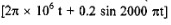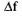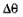# B.TECH 5th sem Analog Communication paper 2016

UniversityVTU, Belgaum, Karnataka
CourseB.TECH
Semester5
SubjectAnalog Communication
Year2016
Downloads1
Uploaded By###### Heman Sharma
100

 Question Marks 0 requests a. Explain mean, correlation and covariance functions of a random process x(t) This question has 0 answers so far. 8 0 requests b. Explain the properties of auto correlation function and power spectral density. This question has 0 answers so far. 12 0 requests a. Define demodulation and show that a square law device can be used to detect AM waves. This question has 0 answers so far. 7 0 requests b. Explain the generation of DSB -SC wave using ring modulator. This question has 0 answers so far. 8 0 requests c. A 250 W carrier of 1000 KHz is simultaneously modulated by sinusoidal signals of 2 KHz,6 KHz and 8 KHz with modulation indices of 35%, 55% and 75% respectively. What are the frequencies present in the modulated wave and what the radiated power?  This question has 0 answers so far. 5 0 requests a. With the neat diagram, explain the operation of quadrature carrier multiplexing. This question has 0 answers so far. 6 0 requests b. Describe phase discrimination method of generating SSB waves. This question has 0 answers so far. 6 0 requests c. Consider a message signal m(t) containing the frequency complents 100, 200 and 400 Hz.This message signal is applied to an SSB modulator together with a carrier at 100 KHz with only USB retained. The coherent detector employed at the receiver uses a local oscillator that gives a sine wave of frequency 100.02 KHz. i) Determine the frequency component of the detector output ii) Repeat your analysis assuming only LSB is transmitted, This question has 0 answers so far. 8 0 requests a. Derive the time domain expression of VSB modulated wave s(t) containing a vestige of upper side band. This question has 0 answers so far. 7 0 requests b. Explain the scheme for generation and demodulation of VSB waves with relevant block diagrams, and mathematical expressions. This question has 0 answers so far. 7 0 requests c. With a neat block diagram, explain the operation of AM super heterodyne receiver. This question has 0 answers so far. 6 0 requests a. Define the terms:i) modulation index This question has 0 answers so far. 2 0 requests a. Define the terms:ii) band width This question has 0 answers so far. 2 0 requests a. Define the terms:iii) frequency deviation in the case of frequency modulation This question has 0 answers so far. 2 0 requests b. Explain the direct method of generating FM waves.  This question has 0 answers so far. 8 0 requests c. An angle modulated signal is defined by s(t) = 10 cosvolts find the following:i) The power in modulated signal ii) The frequency deviationiii) Phase deviationiv) The approximate transmission bandwidth. This question has 0 answers so far. 6 0 requests a. With the help of circuit diagram, explain demodulation of FM wave using balanced frequency discriminator.  This question has 0 answers so far. 8 0 requests b. With relevant block diagram, explain FM stereo multiplexing. This question has 0 answers so far. 6 0 requests c. Explain non-linearity and its effects in FM system. This question has 0 answers so far. 6 0 requests a. Define :shot noise, thermal noise, white noise. This question has 0 answers so far. 6 0 requests b. Define noise equivalent band width and derive the expression for the same. This question has 0 answers so far. 8 0 requests c.Two 2-port devices are connected in cascade. For the first stage, the noise Figure and available power gain are 5 dB and 12 dB respectively. For the second stage, the noise figure and available power gain are 15 dB and 10 dB respectively. Determine the overall noise figure in dB. This question has 0 answers so far. 6 0 requests a. Derive the expression for the figure of merit of DSB-SC receiver. This question has 0 answers so far. 10 0 requests b. Describe the pre-emphasis and de emphasis in FM. This question has 0 answers so far. 6 0 requests c. An FM signal with a deviation of 75 KHz is applied to an FM demodulator. When the input SNR is 15 dB, the modulating frequency is 10 KHz, estimate the SNR at the demodulator output.  This question has 0 answers so far. 4
Chat with us